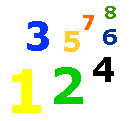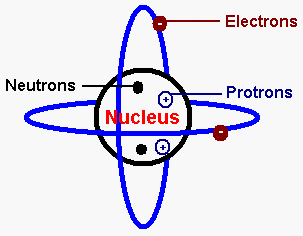# Atomic Weight

Atoms Lesson - Atomic Weight - Atom Mass Number - Science Lessons & Tests - My Schoolhouse - Online Learning

Atoms have charges.  There are two kinds of charges.  There are positive (+) charges and negative (-) charges.  Scientists have discovered that some parts of an atom have positive charges and other parts have negative charges.

PROTONS - have positive charges (+)

ELECTRONS - have negative charges (-)

NEUTRONS - have no charges.  They are neutral.

The number of protons in the nucleus of an atom determines an element's Atomic Number.  An atom has the same number of protons and electrons.  In the diagram below, there are 2 protons and 2 electrons.  The Atomic Number of the element below would be 2.The number of neutrons and protons of an atom is called the atom's Mass Number.

Each PROTON is given a mass of one.

Each NEUTRON is given a mass of one.

Here's an example:

 Name of Element Number of Protons Number of Neutrons Mass Number cobalt 27 32 59

Practice finding the atom's Mass Number for each of the elements listed below.  Remember the atom's  Mass Number equals the sum of the protons and neutrons.

 Name of Element Number of Protons Number of Neutrons Mass Number zinc 30 35 krypton 36 48 potassium 19 20 silver 47 61 lead 82 125 oxygen 8 8 hydrogen 1 0 calcium 20 20

1. A has a positive charge.

2. An  has a negative charge.

3. A has no charge.

4. The number of and protons of an atom is called the atom's Mass Number.